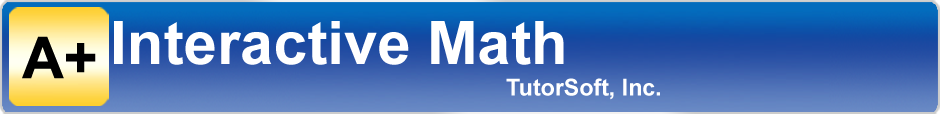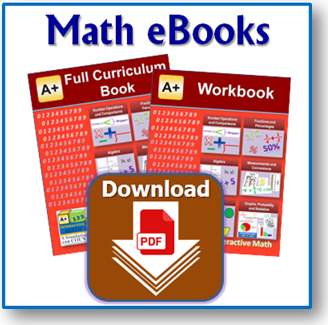## Math eBooks (eTextbooks, eWorkbooks & eSolution Guides)eTextbooks, eWorkbooks & eSolution Guides
A+ Interactive Math provides eTextbooks and eWorkbooks at very affordable prices. eTextbooks and eWorkbooks are great low-cost alternative to printed books as well as online curriculum. eSolution Guides (PDFs on CD) take the guess work out by proving step-by-step solution to each worksheet and chapter test.
Lessons, Practice and Solutions!
Complete curriculum bundle at affordable Prices!

eTextbook
A comprehensive math curriculum book for grades 1-6, Pre-Algebra and Algebra 1 (each grade level sold separately). eTextbook contains easy to understand and fun math lessons that teach various math concepts with appropriate visuals and text
eWorkbook
Practice worksheets, tests and answer keys for all math concepts taught in each grade level. eWorkbook is available for grades 1-6, Pre-Algebra and Algebra 1 where each grade level is sold separately. It allows your student to practice problem solving to master various math concepts that build a solid foundation in math that you can count on.
eSolution Guides (PDFs)
eSolution guide for each problem on the practice worksheets and tests in the eWorkbook provide detailed solution steps with appropriate visuals to each worksheet and test problem to further reinforce math concepts. A PDF for each worksheet and chapter test makes it easy to locate just what you need.

Bring Your Math to Life.
Learn faster. Get better grades. Have more fun. Guaranteed!
Curriculum eBooks
\$9.99
(Reg \$19.99)
Workbooks (eBook)
\$7.99
(Reg \$14.99)
Solution Guides CD
\$9.99
(Reg \$19.99)